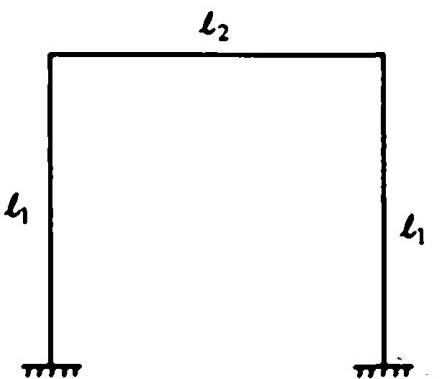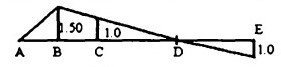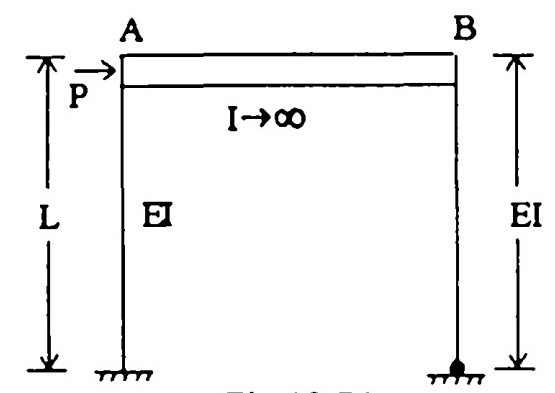Search

# Structural Analysis (141-160)

Updated: Apr 30, 2020

141.For the continuous beam shown in Fig, the influence line diagram for

support reaction at D is best represented as142. Figure shows a portal frame with one end fixed and other hinged.The ratio of the fixed end moments Mʙᴀ/Mᴄᴅ due to sides way will be

1.0

143. Figure shows a portal frame with loads.The bending moment diagram for this frame will be144. Figure 12.65 shows a Pratt trussThe influence line for force in member BC will be145.A two hinged semicircular arch of radius R carries a concentrated load W at the crown. The horizontal thrust is W/π

146.The rigid portal frame shown in Fig. will not have any side sway if I = the moment of inertia of the column cross section

147. For the portal frame shown in Fig.the shear equation is148. Due to some point load anywhere on a fixed beam, the maximum free bending moment is M. The sum of fixed end moment is M

149. A steel frame is shown in Fig.If joint 0 of the frame is rigid, the rotational stiffness of the frame at point O

is given by 11EI/l

150. Consider the following statements : In the beam shown in Fig. , for all

positions of load W (except x = 0)1. bending moment is maximum at B.

2. deflection is zero at A.

3. deflection is zero at B

151. A fixed beam of uniform section is carrying a point load at its mid-span. If the

moment of inertia of the middle half length is now reduced to half its previous

value, then the fixed end moments will increase

152. The maximum bending moment at the left quarter point of a simple b~am due to crossing of UDL of length shorter than the span in the direction left to right; would occur after the load had just crossed the section by

three-fourth of its length

153. When a load crosses a through type Pratt truss in the direction left to right, the nature of force in any diagonal member in the left half of the span would

change from compression to tension

154. In the Fig., x, y and z are the deflections under A, B and C due to loads

P and Q. x', y' and z' are the deflections under A, B and C due to unit load at CPx' + Qy'

155.The influence line R for the beam shown in Fig. 12.72 will be as in156.The moment at the indeterminate support of a two-span continuous beam of 6 m each with simple supports at the ends carrying a udl of 20 kN/m over only the

left span is (flexural rigidity is the same for both the spans)

45 kN.m hogging

157.For the rigid frame shown in Fig. the force required for moving the girder

AB through a horizontal displacement A is given by 15EIΔ/L³

158.For the portal frame shown in Fig., the final end moments are

Mᴀʙ= 15 kN.m

Mʙᴀ= 10 kN.m

Mᴄᴅ = 20 kN.m

The end moment at Mᴅᴄ will be

20 kN.m

159. The slope-deflection equation at the end B of member BC for the frame shown in Fig. will be160.Total degree of indeterminacy (both internal and external) of the plane frame

shown in Fig. is 12